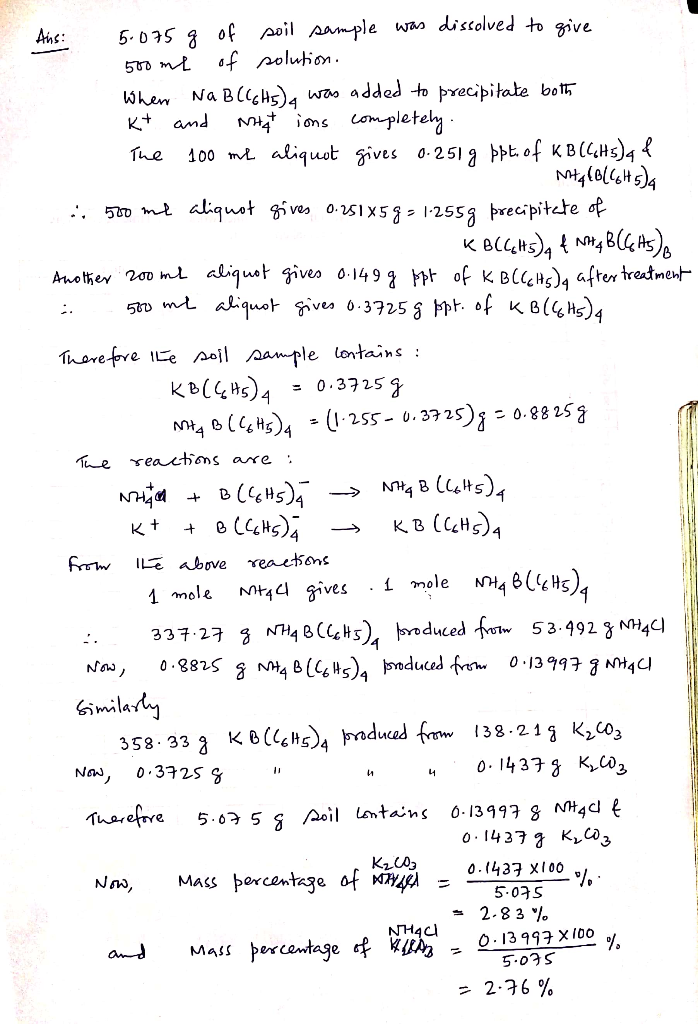# As part of a soil analysis on a plot of land, a scientist wants to determine the ammonium content...

As part of a soil analysis on a plot of land, a scientist wants to determine the ammonium content using gravimetric analysis with sodium tetraphenylborate, Na+B(C6H5)−4.Na+B(C6H5)4−. Unfortunately, the amount of potassium, which also precipitates with sodium tetraphenylborate, is non‑negligible and must be accounted for in the analysis. Assume that all potassium in the soil is present as K2CO3K2CO3 and all ammonium is present as NH4Cl.NH4Cl.

A 5.075 g5.075 g soil sample was dissolved to give 0.500 L0.500 L of solution. A 100.0 mL100.0 mL aliquot was acidified and excess sodium tetraphenylborate was added to precipitate both K+K+ and NH+4NH4+ ions completely.

Compound Formula mass
KB(C6H5)4KB(C6H5)4 358.33 g/mol358.33 g/mol
NH4B(C6H5)4NH4B(C6H5)4 337.27 g/mol337.27 g/mol
K2CO3K2CO3 138.21 g/mol138.21 g/mol
NH4ClNH4Cl 53.492 g/mol53.492 g/mol

B(C6H5)−4+K+⟶KB(C6H5)4(s)B(C6H5)4−+K+⟶KB(C6H5)4(s)

B(C6H5)−4+NH+4⟶NH4B(C6H5)4(s)B(C6H5)4−+NH4+⟶NH4B(C6H5)4(s)

The resulting precipitate amounted to 0.251 g.0.251 g. A new 200.0 mL200.0 mL aliquot of the original solution was made alkaline and heated to remove all of the NH+4NH4+ as NH3.NH3. The resulting solution was then acidified, and excess sodium tetraphenylborate was added to give 0.149 g0.149 gof precipitate.

Find the mass percentages of NH4ClNH4Cl and K2CO3K2CO3 in the original solid.

mass percentage of NH4ClNH4Cl:

%%

mass percentage of K2CO3K2CO3:

%##### Add Answer of: As part of a soil analysis on a plot of land, a scientist wants to determine the ammonium content...
More Homework Help Questions Additional questions in this topic.